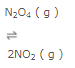Courses

# Dynamic Nature of Equilibrium Class 11 Notes | EduRev

## Class 11 : Dynamic Nature of Equilibrium Class 11 Notes | EduRev

The document Dynamic Nature of Equilibrium Class 11 Notes | EduRev is a part of the Class 11 Course Chemistry Class 11.
All you need of Class 11 at this link: Class 11

Dynamic nature of chemical equilibrium

When the equilibrium is reached, the concentration of each of the reactants and products become constant. In the reaction between H2 and I2 to form HI , the colour becomes constant because the concentration of H2 , I2 and HI becomes constant.

As much of the reactants react to form the products ,the same amount of products react to give back the reactants in the same time. Hence the equilibrium is dynamic in nature and not static.

Experiment

In the Haber’s process ,starting with definite amount of N2 and H2 and carrying out the reaction at a particular temperature, when equilibrium is attained ,the concentration of N2, H2 and NH3 become constant.

If the experiment is repeated by taking deuterium in place of H2 but with the same amounts and exactly similar as before equilibrium is attained containing D2 and ND3in place of H2 and NH3 but in the same amount.

If the two reaction mixtures are mixed ,then after sometime, it is found that the concentration of ammonia and hydrogen are same except that now all forms of ammonia and all forms of hydrogen are present.

This shows that an equilibrium , the reaction is still going on i.e. equilibrium is dynamic in nature.

Characteristics of chemical equilibrium

1) At equilibrium, the concentration of each of the reactants and products becomes constant.

For ex: The constancy of pressure in the evaporation of water , decomposition of calcium carbonate  and constancy of colour at equilibrium in the decomposition of N2O4.

2) At equilibrium the rate of forward reaction becomes equal to that of backward reaction and hence the equilibrium is dynamic in nature.

3) A chemical equilibrium can be established only if none of the products is allowed to escape out or separate out as solids.

4) Chemical equilibrium can be attained from either direction i.e. from the direction of the reactants as well as from the direction of the products.5) A catalyst does not alter the state of equilibrium

A catalyst increases the speed of the forward reaction as well as that of the backward reaction to same extent. Hence, the equilibrium is not disturbed i.e. at equilibrium the concentration of each of the reactant and the product is same as found at equilibrium when no catalyst was added. The only effect of adding the catalyst is that equilibrium is attained quickly.

Offer running on EduRev: Apply code STAYHOME200 to get INR 200 off on our premium plan EduRev Infinity!

## Chemistry Class 11

131 videos|224 docs|178 tests

,

,

,

,

,

,

,

,

,

,

,

,

,

,

,

,

,

,

,

,

,

;